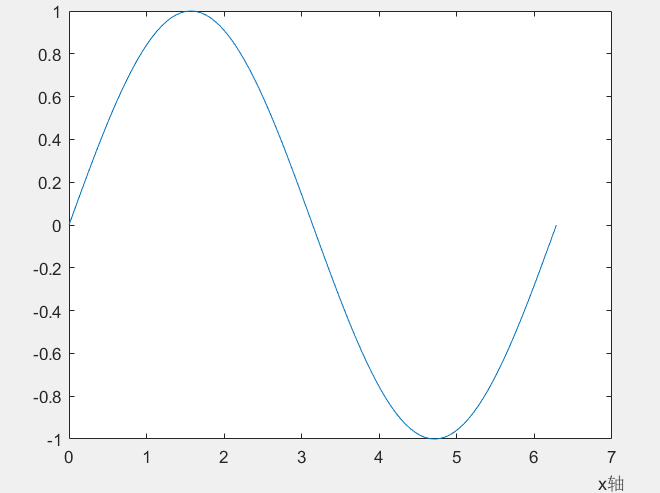• ## matlabxlabel位置设置

千次阅读 2020-03-20 17:54:40
xlabel(‘time’,‘FontSize’,12); 如果没有设置位置，默认是在中间 在xlabel中也有position用法 xlabel(‘time’,‘position’,[900,1870],‘FontSize’,12); 此时‘time’在你设置的位置 还有一种用法是类似图像...
xlabel(‘time’,‘FontSize’,12);
如果没有设置位置，默认是在中间
在xlabel中也有position用法
xlabel(‘time’,‘position’,[900,1870],‘FontSize’,12);
此时‘time’在你设置的位置
还有一种用法是类似图像的用法
pos=axis;%取得当前坐标轴的范围，即[xmin xmax ymin ymax]
xlabel(‘time’,‘FontSize’,12, ‘Position’,[pos(2) pos(3)])
x=0:pi/50:2*pi;
y=sin(x);
plot(x,y);
pos=axis;%取得当前坐标轴的范围，即[xmin xmax ymin ymax]
xlabel('x轴','position',[pos(2) 1.15*pos(3)]);%设置x轴标签的文本在图的右下方，1.15这个值根据自己的需要可以调整
形成的图参考链接2：http://blog.sina.com.cn/s/blog_7e6270010101nt0r.html#commonComment


展开全文• xlabel('步数');ylabel('误差'); % -----------------------------测试样本网络预测 ------------------------ %输入数据归一化 inputn_test=mapminmax( apply',input_test,inputps); [n,m]=size(input_test); ...神经网络 神经网络
• 闲的蛋疼，学学matlab动画，三体运动还是有点问题(提示line没xdate属性，脑瓜...axis equalgrid onhold oncolordef blackview(3)xlabel('x')ylabel('y')zlabel('z')hold on%初始化参数设置(质量、初始位置、初始速度...
闲的蛋疼，学学matlab动画，三体运动还是有点问题(提示line没xdate属性，脑瓜也疼了)，不多废话上代码clc;clear;close;figure('name','三体运动');axis equalgrid onhold oncolordef blackview(3)xlabel('x')ylabel('y')zlabel('z')hold on%初始化参数设置(质量、初始位置、初始速度、万有引力常数、时间步长)m1=rand()*10^23;m2=rand()*10^23;m3=rand()*10^23;pos1=[rand()*10^7,rand()*10^7,rand()*10^7];pos2=[rand()*10^7,rand()*10^7,rand()*10^7];pos3=[rand()*10^7,rand()*10^7,rand()*10^7];v1=[rand()*1000,rand()*1000,rand()*1000];v2=[rand()*1000,rand()*1000,rand()*1000];v3=[rand()*1000,rand()*1000,rand()*1000];G=6.67*10^(-11);dt=0.005;%行星和轨迹(定义行星位置和轨迹变量)planet1=plot3(pos1(1),pos1(2),pos1(3),'b:.','markersize',20);planet2=plot3(pos2(1),pos2(2),pos2(3),'r:.','markersize',20);planet3=plot3(pos3(1),pos3(2),pos3(3),'y:.','markersize',20);h1=animatedline('color','b');h2=animatedline('color','r');h3=animatedline('color','y');frame=0;while true%不断循环计算距离、相互作用力、速度、加速度、位置r12=normest(pos2-pos1);r23=normest(pos3-pos2);r13=normest(pos3-pos1);F12_val=G*m1*m2/(r12^2);F23_val=G*m2*m3/(r23^2);F13_val=G*m1*m3/(r13^2);F12_dir=(pos2-pos1)/normest(pos2-pos1);F23_dir=(pos3-pos2)/normest(pos3-pos2);F13_dir=(pos3-pos1)/normest(pos3-pos1);a1=((F12_val)*(F12_dir)+(F13_val)*(F13_dir))/m1;a2=((-F12_val)*(F12_dir)+(-F23_val)*(F23_dir))/m2;a3=((-F13_val)*(F13_dir)+(-F23_val)*(F23_dir))/m3;v1=v1+a1*dt;v2=v2+a2*dt;v3=v3+a3*dt;pos1=pos1+v1*dt+1/2*a1*dt^2;pos2=pos2+v2*dt+1/2*a2*dt^2;pos3=pos3+v3*dt+1/2*a3*dt^2;frame=frame+1;if frame==10000frame=0;% set(planet1,'position',[pos1(1),pos1(2),pos1(3)]);%set(planet3,'position',pos3(1),'YData',pos3(2),'ZData',pos3(3));addpoints(h1,pos1(1),pos1(2),pos1(3));addpoints(h2,pos2(1),pos2(2),pos2(3));addpoints(h3,pos3(1),pos3(2),pos3(3));drawnowendif r12==0||r23==0||r13==0       %判断是否相撞breakendend好吧很丑标签：rand,10,三体,dt,matlab,运动,pos2,pos3,pos1来源： https://blog.csdn.net/JISANSAN/article/details/100117190
展开全文• matlab绘图基础%曲线图，描点连线： x=linspace(0,2*pi,30); y=sin(x); z=cos(x); plot(x,y,'r',x,z,'--')%图形处理 %图形标注： title('sin(x)和cos(x)的曲线') xlabel('X轴') ylabel('y轴') %text(x,y,字符串)在xy...
matlab绘图基础

%曲线图，描点连线：
x=linspace(0,2*pi,30);
y=sin(x);
z=cos(x);
plot(x,y,'r',x,z,'--')

%图形处理
%图形标注：
title('sin(x)和cos(x)的曲线')
xlabel('X轴')
ylabel('y轴')
%text(x,y,字符串)在xy处加注文本
gtext('y1=sin(x)')%使用鼠标指定位置加标注
gtext('y2=cos(x)')
legend('sin(x)','cos(x)')%添加样例标注
grid on %添加网格
hold on %保持当前图形 在其上继续绘图
%定制坐标axis([xmin xmax ymin ymax zmin zmax])
%绘制多个图形figure,在第二个图形之前加个figure(2),第三个图形加figure(3)
%subplot（m,n,p）讲作图区域划分成m*n块，p为在第几块工作
%缩放图形zoom on，zoom off关闭缩放模式
%改变视角view(a,b)

%符号函数（显函数，隐函数，参数方程）ezplot
%ezplot('f(x)',[xmin,xmax])
%ezplot('f(x,y)',[xmin,xmax,ymin,ymax])
%ezplot('x(t)','y(t)',[tmin,tmax])
ezplot('sin(x)',[0,pi])
ezplot('exp(x)+sin(x*y)',[-2,0.5,0,2])
ezplot('cos(t)^3','sin(t)^3',[0,2*pi])

%对数坐标图，分别画出y=x^3函数，对数，半对数坐标图
x=[0:1:100]

%函数图
subplot(231)  %subplot(mnp)在一个界面输出两行三列图形，p为第几个图
plot(x,x.^3)
grid on %加上网格线
title 'plot-y=x^3'

%两边取对数
subplot(232)
loglog(x,x.^3)
grid on
title 'loglog-logy=3logx'

%x轴对数转换
subplot(233)
semilogx(x,x.^3)
grid on %添加网格
title 'semilogx-y=3logx'

%y轴对数转换
subplot(234)
semilogy(x,x.^3)
grid on
title 'semilogy-logy=x^3'

%双坐标输出
subplot(235)
plotyy(x,x.^3,x,x)
grid on
title 'plotyy-y=x^3,y=x'

%一条曲线plot(x,y,z,s)xyz为n维向量，s为指定颜色
t=0:pi/50:10*pi
plot3(sin(t),cos(t),t)
rotate3d%旋转
%多条曲线，，，略

%空间曲面
%（1）surf（x,y,z）xyz横坐标纵坐标函数值
x=-3:0.1:3;
y=1:0.1:5;
[x,y]=meshgrid(x,y)%产生两个矩阵，一个以x为行，另一个以y为列
z=(x+y).^2
surf(x,y,z)
%(2)画网格图，图形为网格，将surf换成mesh即可
x=-3:0.1:3
y=1:0.1:5
[x,y]=meshgrid(x,y)
z=(x+y).^2
mesh(x,y,z)
%带底座的网格图meshz
[x,y]=meshgrid(-3:.125:3)
z=peaks(x,y)%生成一个测试图形
meshz(x,y,z)

%特殊的二维图
%polar(thera,rho,s)thera表示弧度，rho为极半径，s为指定线型
theta=linspace(0,2*pi),
rho=sin(2*theta).*cos(2*theta);
polar(theta,rho,'g')
title('Polar plot of sin(2*theta).*cos(2*theta)');
%散点图scatter(X,Y)X和Y是数据向量，以X中数据为横坐标，以Y中数据位纵坐标描绘散点图，点的形状默认使用圈。
%等高线contour (x,y,z,n)
%特殊三维图：等高线，散点图，，，，，，暂略

展开全文• 本文用来记录在matlab绘图过程中，常用的指令，仅供大家参考，不定时更新。 指定标题的位置及大小： title(‘txt’,‘position’,[-120,0],‘FontSize’,16); 坐标轴用Latex解释器： xlabel({time /μ\muμs’},...
本文用来记录在matlab绘图过程中，常用的指令，仅供大家参考，不定时更新。
指定标题的位置及大小：
title(‘txt’,‘position’,[-120,0],‘FontSize’,16);
坐标轴用Latex解释器：
xlabel({time /$\mu$s’},‘Interpreter’,‘latex’);
插入图例：
legend(‘legend1’,‘legend2’);
插入文本，支持latex:
text(0,120,’\mus’);


展开全文• clear all; x=(0:0.1:2*pi)'; y1=2*exp(-0.5*x)*[1,-1]; y2=2*exp(-0.5*x).*sin(2*pi*x); x1=(0:12)/2; y3=2*exp(-0.5*x1).*sin(2*pi*x1); plot(x,y1,x,y2,x1,y3);...%窗口顶端中间位置标题 xlabel('变量X');%X轴...
• matlab中二维线画图函数 1. plot(x,y); %x y 为相应点集 2.plot(x,y1,x,y2); % 在一个窗口下绘制多条曲线之方法一 3.hold on %在一个窗口下绘制多条曲线之方法二 plot(x,y1); plot(x,y2); hold off 4.plot...
• MATLAB为什么绘制的仿真数据曲线图像为什么总是模糊、不清晰呢...首先谈谈MATLAB绘制的函数图像几个实用的函数：xlabel % 标记x轴ylabel % 标记y轴set(gcf,’position’,[100 200 400 300]) % 设置当前figure位置(1...
• 闲的蛋疼，学学matlab动画，三体运动还是有点问题（提示line没xdate属性，算了所幸直接注释掉了，就是更新一下位置，前面已经计算了，脑瓜也疼了），不多废话上代码 clc;clear;close; figure('name','三体运动'); ...
• MATLAB为什么绘制的仿真数据曲线图像为什么总是模糊、不清晰呢...首先谈谈MATLAB绘制的函数图像几个实用的函数：xlabel % 标记x轴ylabel % 标记y轴set(gcf,’position’,[100 200 400 300]) % 设置当前figure位置(1...
• ## 遗传算法MATLAB代码

热门讨论 2012-11-08 20:19:48
xlabel('x');ylabel('f(x)');%标记轴 % 迭代前的初始化 vmfit=[];%平均适应度 vx=[]; %最优适应度 it=1; % 迭代计数器 % 开始进化 while it迭代次数 0代 %Reproduction(Bi-classist Selection) vtemp=roulette(v,...遗传算法
• text对象可以通过title,xlabel,ylabel,zlabel或text函数创建。 texlabel用于转换MATLAB表达式为Tex格式字符串。 texlabel(f) Tex字符还可以设置字体，颜色和位置。 texlabel(0.5,0.5,'\bf\...
• 指定坐标添加标记: text(x,y,str) 手动指定位置添加标记: gtext(str) 横纵坐标 xlabel('')%横 ylabel('')%纵 tittle('')%标题
• y = gaussmf(x,[sig c]) 其中，c是位置参数，sig是尺度参数，控制图形的胖瘦。x = 0:0.1:10; y = gaussmf(x,[2 5]); plot(x,y) xlabel('gaussmf, P=[2 5]')参考
• 最近画的一幅图的X轴是时间，需要标注月份、日期和时分，显然每个xtick...根据xticks的位置和y轴的范围设置两行text的范围，最后下移xlabel的范围。 画完之后又发现colorbar太宽了，看起来不美观。结果调整colorbar pos
• 开始工作：绘图举例 %Matlab内快速绘图fplot(@(x) sin(2*pi*x)); % 给图形添加基本注释信息 title('sin(2*pi*x)');...第二步：通过gcf句柄获取当前图片的位置信息，位置信息由4维的向量描述，即pos=...python java 计算机视觉 机器学习
• 开始工作：绘图举例 %Matlab内快速绘图fplot(@(x) sin(2*pi*x)); % 给图形添加基本注释信息 title('sin(2*pi*x)'); xlabel('x');...第二步：通过gcf句柄获取当前图片的位置信息，位置信息由4维的向量...
• clf;load( 'C:\Users\robot\...figure(1),plot3(pose2(:, 4), pose2(:,8), pose2(:, 12)) % 绘制位置三维曲线，列标号4、8、12表示位置坐标值 view(0,0) % 设置观察视角为“观察x-z平面” title( '路线1' ) xlabel( 'xVO
• plot函数的xlabel、ylabel、title、text、legend1、在图像某个位置显示文字信息，2、用text()显示plot函数的坐标点;3、用legend()在一张图同时显示不同曲线;4、用xlabel()、ylabel()、title()显示横纵坐标和标题;5、...图像处理 plot
• ## Matlab图像标题_title

千次阅读 2015-04-14 09:19:41
xlabel('x')：图像下方加标题x；title：实现图像任意位置加标题。   Example：实现任意个数图像且携带任意标题（应用绿色循环实现多个图像同时显示）   close all;  for i=1:10  figure(i);  x = -pi:...
• 问题来源 绘制实验数据是，想像部分...1 matlab中subplot子图使用title函数 title('(a)','position',[-4,38],'FontSize',16); 指定title的位置即可 2 使用xlabel函数 直接使用xlabel函数 对子图进行编号 3 使用tex...
• clear； clc; I=imread('C:\Users\dell\Desktop\gear2.jpg');%%从桌面路径显示图片 subplot(2,3,1),imshow(I);%设置了一个两行三列的位置，让这个图片显示在第一个位置 xlabel('(a)原图');%在X方向上显示这段文字计算机视觉
• 其中，c是位置参数，sig是尺度参数，控制图形的胖瘦。 x = 0:0.1:10; y = gaussmf(x,[2 5]); plot(x,y) xlabel('gaussmf, P=[2 5]') ylabel('gaussmf') legend('gaussmf'); %添加图例代码 结果图 ...绘制
• 求助非矢量型的怎么写 自己大概写的如下 是错的 因为不知道H的位置和表达方式 to=cputime(); x = load('ex4x.dat'); y = load('ex4y.dat'); m=length(y); x = [ones(m, 1), x]; pos = find(y); neg = find(y == 0)...
• 在text对象的函数中（函数title、xlabel、ylabel、zlabel或text），说明文字除使用标准的ASCII字符外，还可使用LaTeX格式的控制字符，这样就可以在图形上添加希腊字母、数学符号及公式等内容。例如，text(0.3,0.5,...text
• 经常听有人说想画 “4D图形”，前3维数据[x,y,z]表示空间位置，第4维数据v表示颜色（温度等），这类图形可以方便地通过slice切片实现： slice，这里就是在指定的切片上在空间坐标[x,y,z]处，用v值指定颜色画图。...
• xlabel, ylabel, zlabel; title axis equal hold off disp disp) %排除重复的点复制代码经测试十几种奇怪的曲线相交（包括参数方程形式的曲线），目前发现上述代码的方法有四种情况会出现遗漏一两个交点。（其实上面...
• xlabel('x(m)'); ylabel('y(m)'); title('DWA算法（α=0.1，β=0.2，r=0.2）') set(gca,'xtick',[-1:1:11]); set(gca,'ytick',[-1:1:11]); %******* grid on; drawnow; %movcount=movcount+1; ...自动驾驶# matlabxlabel位置matlab 订阅